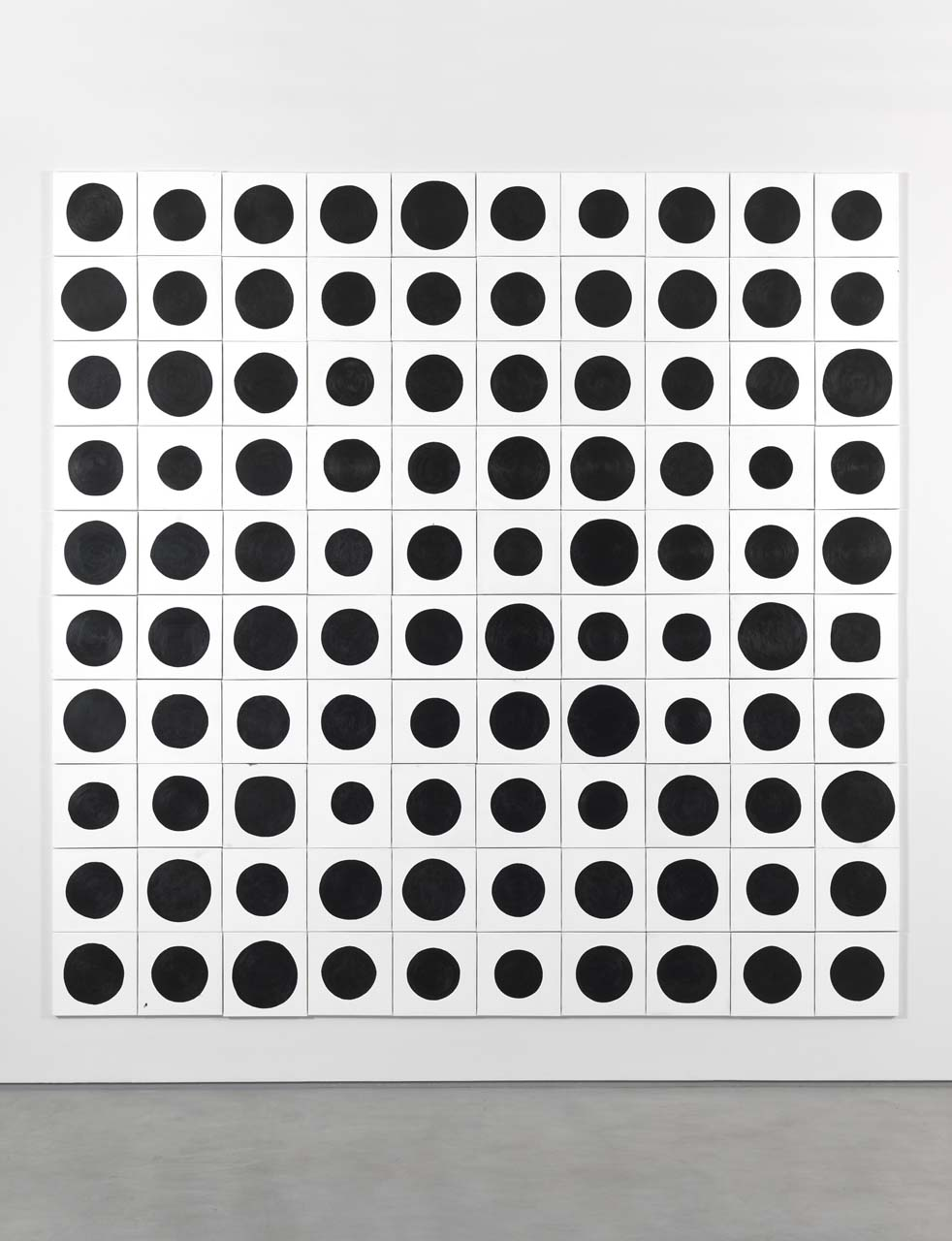#### Howdy, Stranger!

We are about to switch to a new forum software. Until then we have removed the registration on this forum.

# How can I make a grid of independent objects?

edited March 2017

So I wanted to replicate this photo, a grid of circles with changing random values:But i can't see to figure out how to make the grid. I know i can add parameters into the new object, but can't figure out how. I know we can use loops for the y and the x, but i'm not nailing it. Here's the code I have so far:

``````var blobs = [];

function setup() {
createCanvas(400, 400);
frameRate (10);
for (var i = 0; i < 100; i++) {
blobs[i] = new Blob();
}
}

function draw() {
background(0);
for (var i = 0; i < blobs.length; i++) {
blobs[i].display(); blobs[i].move();
}
}

function Blob() {
this.x = width/2;
this.y = height/2;
this.size = 50;
this.extra = 1;

this.display = function() {
noStroke();
fill(255, 0, 0,100);
ellipse(this.x, this.y, this.size + random(-this.extra, this.extra), this.size + random(-this.extra, this.extra));
}

this.move = function() {
this.x = this.x + random(-1, 1);
this.y = this.y + random(-1, 1);
}
}
``````
Tagged:

• Edit post, highlight code, press Ctrl-o to format.

Each blob already has an x and a y, you need to calculate it for each one based on its position on the list. You can pass this in via the constructor.

Ie, use new Blob(i)

• You'll need to change the constructor to match but format the code first (that way we can quote line numbers)

• Check this post.

******EDIT: I jsut notice this is p5.js. The concept in the next post is about the same.

https://forum.processing.org/two/discussion/21231/line-by-line-grid-my-sketch-does-not-start-properly#latest

kf

• I have edited the code

• As koogs suggested, you need to change the constructor of your object:

``````function Blob(xx,yy) {
this.x = xx;
this.y = yy;

ANY OTHER CODE BELOW

}
``````

Then you need to add these next global variables:

``````    var ncols=10;
var nrows=5;
``````

And changed your setup function for this:

``````    function setup() {
createCanvas(400, 400);
frameRate (10);
for (var i = 0; i < nrows; i++) {
for (var j = 0; j < ncols; j++) {
let x=j*width/(ncols*1.0);
let y=i*height/(nrows*1.0);
blobs[i*ncols+j] = new Blob(x,y);
}
}
}
``````

Kf

• edited March 2017 Answer ✓

so, in Blob, add a constructor that takes two parameters, x and y

set this.x to the passed-in x, this.y to the passed-in y

something like changing line 18 to

``````function Blob(x, y) {
``````

and replacing width / 2 and height / 2 with x and y respectively in lines 19, 20

and now you have to add those parameters to you call to new Blob in line 7...